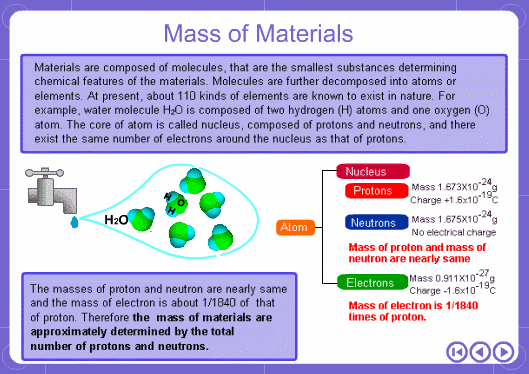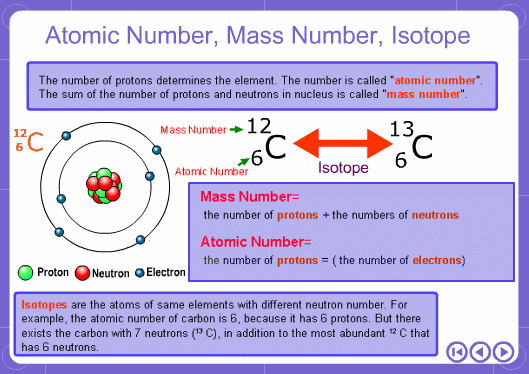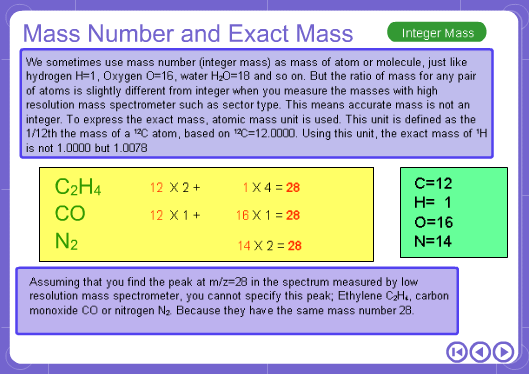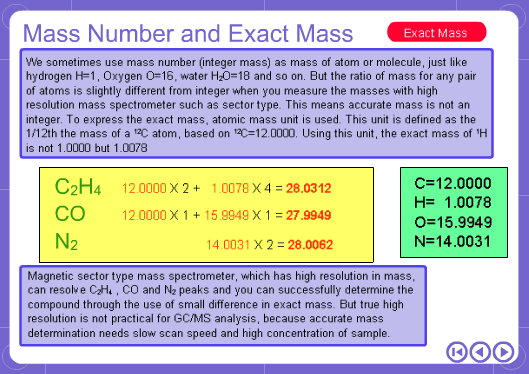# Why are there decimal points in MS data?

## 1. BASIC MASS SPECTROMETRY

### Why are there decimal points in MS data?

The mass numbers used in mass analysis are defined as follows. The mass number of the carbon 12 isotope, consisting of 6 protons and 6 neutrons, is defined as the integer 12. A fraction of 1/12 of the mass of carbon 12 is defined as a mass unit. When measured using this unit the mass number of hydrogen atoms is 1.007825, the mass number of nitrogen atoms is 14.003074, and the mass number of oxygen atoms is 15.9949146. Organic compounds consist of carbon, hydrogen, nitrogen, oxygen, and other elements. Therefore, the mass numbers of molecules, strictly speaking, always have numbers after the decimal place. When molecular weights are small, the variation from the integer is small and the values are often represented as the nearest integer.Back to Index